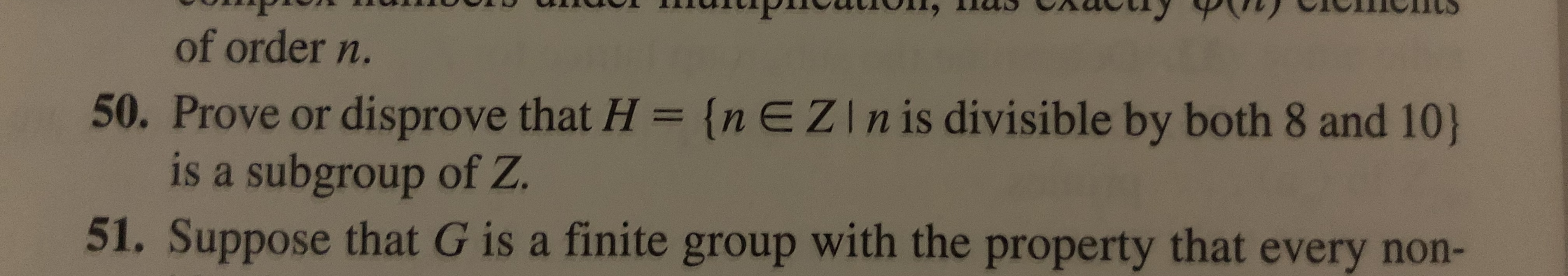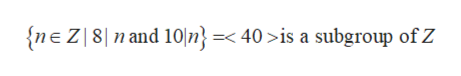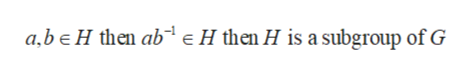of order n.50. Prove or disprove that H = {nEZIn is divisible by both 8 and 10)is a subgroup of Z.51. Suppose that G is a finite group with the property that every non-

Question

50help_outlineImage Transcriptioncloseof order n. 50. Prove or disprove that H = {nEZIn is divisible by both 8 and 10) is a subgroup of Z. 51. Suppose that G is a finite group with the property that every non- fullscreen
Step 1

From the given information, it is needed to prove or disprove that H is a subgroup of Z:

Step 2

A number n € Z is divisible by both 8 and 10 if and only if it is divisible by lcm (8,10) = 40 that is:help_outlineImage Transcriptionclosen and 10n} =< 40 >is a subgroup of Z {neZ8 fullscreen
Step 3

Now, to prove that <40> is a subgroup of Z.

To show it is a ...help_outlineImage Transcriptionclosea,be H then ab eH then H is a subgroup of G fullscreen

Want to see the full answer?

See Solution

Want to see this answer and more?

Our solutions are written by experts, many with advanced degrees, and available 24/7

See Solution
Tagged in

Math